• ## 反向传播

千次阅读 多人点赞 2019-05-18 22:58:54
BP算法是个迭代算法，它的基本思想如下： 将训练集数据输入到神经网络的输入层，经过隐藏层，最后达到输出层并输出结果，这就是前向传播过程。 由于神经网络的输出结果与实际结果有误差，则计算估计值与实际值...
简介
误差反向传播算法简称反向传播算法(Back Propagation)。使用反向传播算法的多层感知器又称为BP神经网络。
BP算法是一个迭代算法，它的基本思想如下：

将训练集数据输入到神经网络的输入层，经过隐藏层，最后达到输出层并输出结果，这就是前向传播过程。
由于神经网络的输出结果与实际结果有误差，则计算估计值与实际值之间的误差，并将该误差从输出层向隐藏层反向传播，直至传播到输入层；
在反向传播的过程中，根据误差调整各种参数的值（相连神经元的权重），使得总损失函数减小。
迭代上述三个步骤（即对数据进行反复训练），直到满足停止准则。

示例
有如下一个神经网络：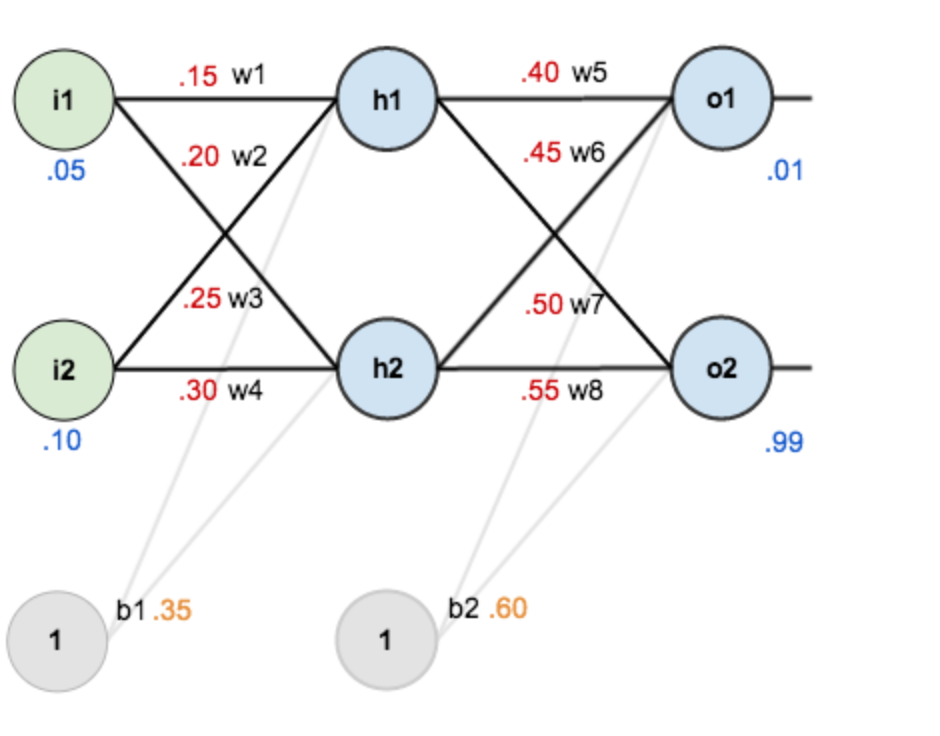第一层是输入层，包含两个神经元 $i_1$，$i_2$ 和偏置项 $b_1$；第二层是隐藏层，包含两个神经元 $h_1$，$h_2$ 和偏置项 $b_2$；第三层是输出 $o_1$，$o_2$。每条线上标的 $w_i$ 是层与层之间连接的权重。激活函数是 $sigmod$ 函数。我们用 $z$ 表示某神经元的加权输入和；用 $a$ 表示某神经元的输出。
上述各参数赋值如下：

参数
值

$i_1$
0.05

$i_2$
0.10

$w_1$
0.15

$w_2$
0.20

$w_3$
0.25

$w_4$
0.30

$w_5$
0.40

$w_6$
0.45

$w_7$
0.50

$w_8$
0.55

$b_1$
0.35

$b_2$
0.60

$o_1$
0.01

$o_2$
0.99

Step 1 前向传播
输入层 —> 隐藏层
神经元 $h_1$ 的输入加权和：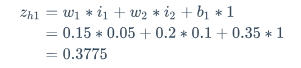神经元 $h_1$ 的输出 $a_{h1}$ ：
$a_{h1} = \frac{1}{1+e^{-z_{h1}}} = \frac{1}{1+e^{-0.3775}} = 0.593269992$
同理可得，神经元 $h_2$ 的输出 $a_{h2}$ ：
$a_{h2} = 0.596884378$
隐藏层 —> 输出层
计算输出层神经元 $o1$ 和 $o2$ 的值：前向传播的过程就结束了，我们得到的输出值是 $[0.751365069, 0.772928465]$ ，与实际值 $[0.01, 0.99]$ 相差还很远。接下来我们对误差进行反向传播，更新权值，重新计算输出。
Step 2 反向传播

计算损失函数：

$E_{total} = \sum\frac{1}{2}(target - output)^2$
但是有两个输出，所以分别计算 $o_1$ 和 $o_2$ 的损失值，总误差为两者之和：
$E_{o_1} = \frac {1}{2}(0.01 - 0.751365069)^2 = 0.274811083 \\ E_{o_2} = \frac {1}{2}(0.99 - 0.772928465)^2 = 0.023560026 \\ E_{total} = E_{o_1} + E_{o_2} = 0.274811083 + 0.023560026 = 0.298371109$

隐藏层 —> 输出层的权值更新

以权重参数 $w_5$ 为例，如果我们想知道 $w_5$ 对整体损失产生了多少影响，可以用整体损失对 $w_5$ 求偏导：
$\frac{\partial E_{total}}{\partial w_5} = {\frac {\partial E_{total}}{\partial a_{o_1}}}*{\frac {\partial a_{o_1}}{\partial z_{o_1}} }*{ \frac {\partial z_{o_1}} {\partial w_5} }$
下面的图可以更直观了解误差是如何反向传播的：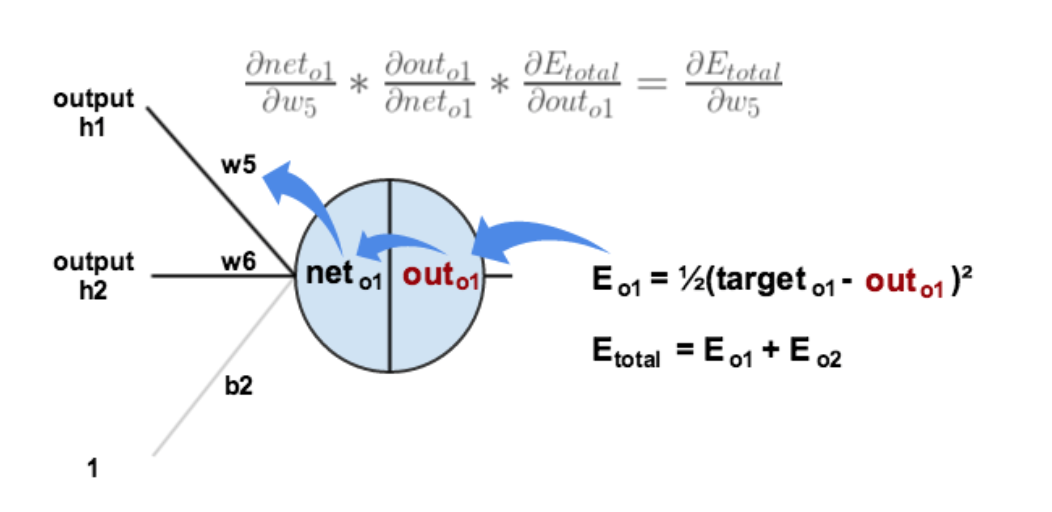我们现在分别来计算每个式子的值：
计算 $\frac {\partial E_{total}} {\partial a_{o_1}}$ ：
$E_{total} = \frac {1}{2}(target_{o_1} - a_{o_1})^2 + \frac {1}{2}(target_{o_2} - a_{o_1})^2 \\ \frac {\partial E_{total}} {\partial a_{o_1}} = 2 * \frac {1}{2} (target_{o_1} - a_{o_1})*-1 \\ \frac {\partial E_{total}} {\partial a_{o_1}} = -(target_{o_1} - a_{o_1}) = 0.751365069-0.01=0.741365069 \\$
计算 $\frac {\partial E_{total}} {\partial a_{o_1}}$ ：
$a_{o_1} = \frac {1}{1+e^{-z_{o_1}}} \\ \frac {\partial a_{o_1}} {\partial z_{o_1}} = a_{o_1}*(1-a_{o_1}) = 0.751365069*(1-0.751365069) = 0.186815602$
计算 $\frac {\partial z_{o_1}} {\partial w_5}$ ：
$z_{o_1} = w_5*a_{h1} + w_6*a_{h2} + b_2*1 \\ \frac {\partial z_{o_1}} {\partial w_5} = a_{h_1} = 0.593269992$
最后三者相乘：
$\frac {\partial E_{total}} {\partial w_5} = 0.741365069*0.186815602*0.593269992 = 0.082167041$
这样我们就算出整体损失 $E_{total}$ 对 $w_5$ 的偏导值。
$\frac {\partial E_{total}} {\partial w_5} = -(target_{o_1} - a_{o_1}) * a_{o_1}*(1-a_{o_1}) * a_{h_1}$
针对上述公式，为了表达方便，使用 $\delta_{o_1}$ 来表示输出层的误差：
$\delta_{o_1} = {\frac {\partial E_{total}}{\partial a_{o_1}}}*{\frac {\partial a_{o_1}}{\partial z_{o_1}} } = \frac {\partial E_{total}} {\partial z_{o_1}} \\ \delta_{o_1} = -(target_{o_1} - a_{o_1}) * a_{o_1}*(1-a_{o_1})$
因此整体损失 $E_{total}$ 对 $w_5$ 的偏导值可以表示为：
$\frac {\partial E_{total}}{\partial w_5} = \delta_{o_1}*a_{h_1}$
最后我们来更新 $w_5$ 的值：
$w_5^+ = w_5 - \eta * \frac {\partial E_{total}} {\partial w_5} = 0.4 - 0.5*0.082167041 = 0.35891648 \qquad \eta: 学习率$
同理可更新 $w_6, w_7, w_8$ ：
$w_6^+ = 0.408666186 \\ w_7^+ = 0.511301270 \\ w_8^+ = 0.561370121$

隐藏层 —> 隐藏层的权值更新：

计算 $\frac {\partial E_{total}} {\partial w_1}$ 与上述方法类似，但需要注意下图：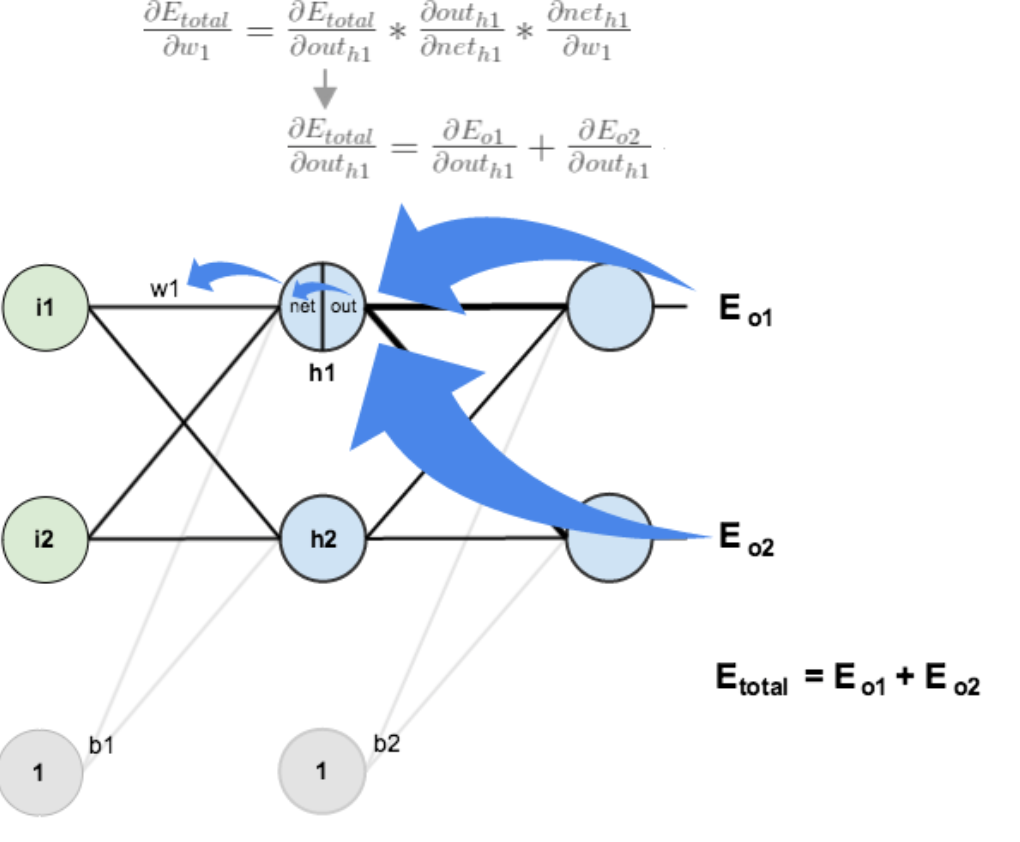计算 $\frac {\partial E_{total}} {\partial a_{h_1}}$ ：
$\frac {\partial E_{total}} {\partial a_{h_1}} = \frac {\partial E_{o_1}} {\partial a_{h_1}} + \frac {\partial E_{o_2}} {\partial a_{h_1}}$
先计算 $\frac {\partial E_{o_1}} {\partial a_{h_1}}$ ：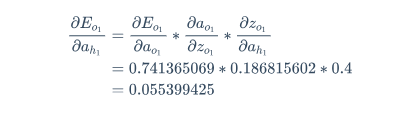同理可得：
$\frac {\partial E_{o_2}} {\partial a_{h_1}} = -0.019049119$
两者相加得：
$\frac {\partial E_{total}} {\partial a_{h_1}} = 0.055399425 - 0.019049119 = 0.036350306$
计算 $\frac {a_{h_1}} {z_{h_1}}$ ：
$\frac {a_{h_1}} {z_{h_1}} = a_{h_1} * (1-a_{h_1}) = 0.593269992*(1-0.593269992) = 0.2413007086$
计算 $\frac {\partial z_{h_1}} {\partial w_1}$
$\frac {\partial z_{h_1}} {\partial w_1} = i_1 = 0.05$
最后三者相互乘：
$\frac {\partial E_{total}} {\partial w_1} = 0.036350306 * 0.2413007086 * 0.05 = 0.000438568$
为了简化公式，用 $\delta_{h_1}$ 表示隐藏层单元 $h_1$ 的误差：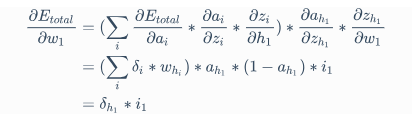最后更新 $w_1$ 的权值：
$w_1^+ = w_1 - \eta * \frac {\partial E_{total}} {\partial w_1} = 0.15 - 0.5*0.000438568 = 0.149780716$
同理，更新 $w_2, w_3, w_4$ 权值：
$w_2^+ = 0.19956143 \\ w_3^+ = 0.24975114 \\ w_4^+ = 0.29950229$
这样，反向传播算法就完成了，最后我们再把更新的权值重新计算，不停地迭代。在这个例子中第一次迭代之后，总误差 $E_{total}$ 由0.298371109下降至0.291027924。迭代10000次后，总误差为0.000035085，输出为$[0.015912196,0.984065734](原输入为[0.01,0.99]$ ，证明效果还是不错的。
公式推导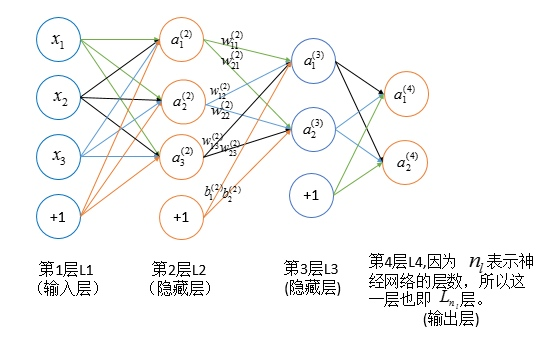符号说明

符号
说明

$n_l$
网络层数

$y_j$
输出层第 $j$ 类标签

$S_l$
第 $l$ 层神经元个数（不包括偏置项）

$g(x)$
激活函数

$w_{ij}^{l}$
第 $l-1$ 层的第 $j$ 个神经元连接到第 $l$ 层第 $i$ 个神经元的权重

$b_i^{l}$
第 $l$ 层的第 $i$ 个神经元的偏置

$z_i^{l}$
第 $l$ 层的第 $i$ 个神经元的输入加权和

$a_i^{l}$
第 $l$ 层的第 $i$ 个神经元的输出（激活值）

$\delta_i^{l}$
第 $l$ 层的第 $i$ 个神经元产生的错误

推导过程
基本公式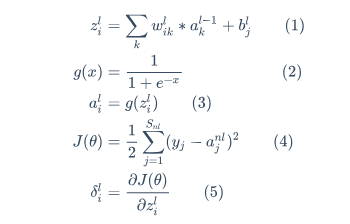梯度方向传播公式推导
初始条件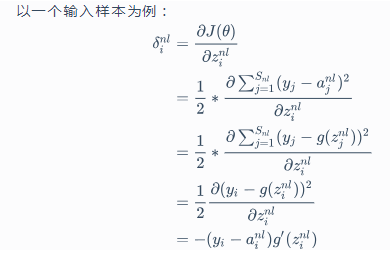递推公式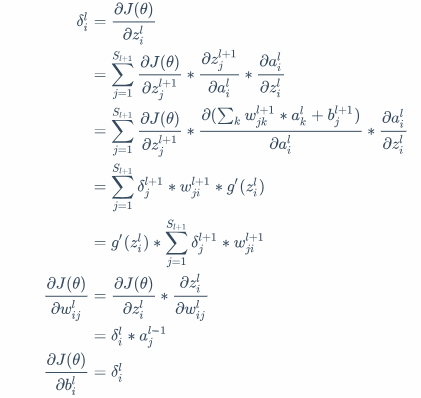反向传播伪代码

输入训练集。
对于训练集的每个样本 $\vec x$ ，设输入层对应的激活值为 $a^l$ ：

前向传播：$z^l = w^l*a^{l-1}+b^l, a^l = g(z^l)$
计算输出层产生的误差：$\delta^L = \frac {\partial J(\theta)} {\partial a^L} \odot g'(z^L)$
反向传播错误：$\delta^l = ((w^{l+1})^T*\delta^{l+1}) \odot g'(z^l)$

使用梯度下降训练参数：

$w^l \dashrightarrow w^l - \frac {\alpha} {m} \sum_x\delta^{x, l}*(a^{x, l-1})^T$
$b^l \dashrightarrow b^l - \frac {\eta} {m} \sum_x\delta^{x, l}$

交叉熵损失函数推导
对于多分类问题，$softmax$ 函数可以将神经网络的输出变成一个概率分布。它只是一个额外的处理层，下图展示了加上了 $softmax$ 回归的神经网络结构图：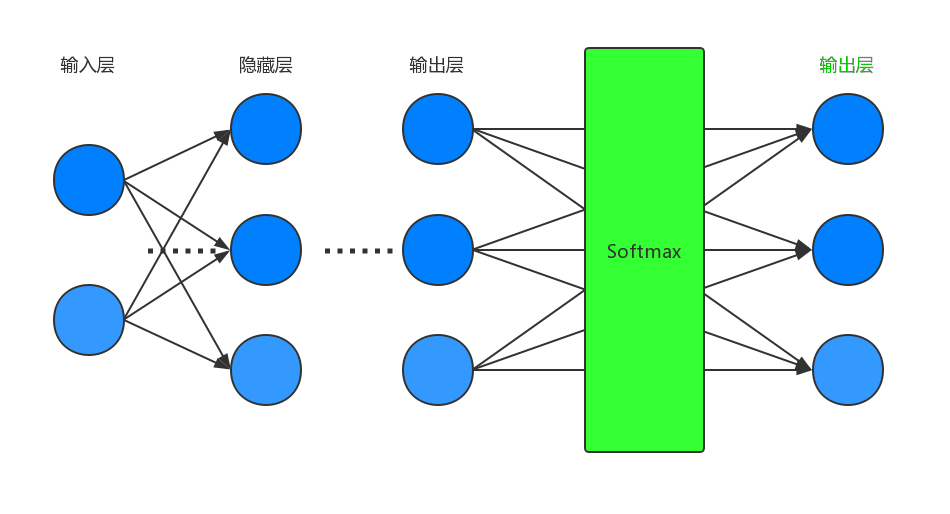递推公式仍然和上述递推公式保持一致。初始条件如下：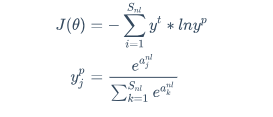$softmax$ 偏导数计算：
\frac {\partial y_j^p} {\partial a_i^{nl}} = \left\{ \begin{aligned} -y_i^p*y_j^p \qquad i \neq j \\ y_i^p*(1-y_i^p) i = j \end{aligned} \right.
推导过程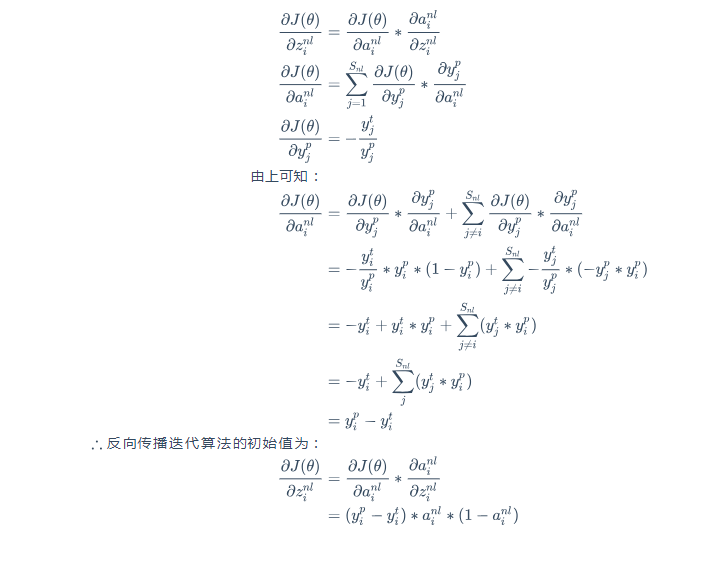参考自：

https://www.cnblogs.com/charlotte77/p/5629865.html?tdsourcetag=s_pcqq_aiomsg
https://blog.csdn.net/u014313009/article/details/51039334
https://www.cnblogs.com/nowgood/p/backprop2.html?tdsourcetag=s_pcqq_aiomsg


展开全文• 、引言 从Nginx入门学习开始、到现在所讲的Nginx反向代理。我们的Nginx学习已经进入白热化状态，前面所学只是铺垫，真正在公司的业务...反向代理流程 话说这个Nginx反向代理+负载均衡难吗？实话告诉你们 ...
一、引言

从Nginx入门学习开始、到现在所讲的Nginx反向代理。我们的Nginx学习已经进入白热化状态，前面所学只是铺垫，真正在公司的业务场景中Nginx绝大数用来反向代理+负载均衡所用。相信大家在学习Nginx之前对反向代理和负载均衡就有所闻知，那么今天小编带领大家先来体验一下使用这个Nginx反向代理的感觉。

二、反向代理流程

话说这个Nginx反向代理+负载均衡难吗？实话告诉你们 ：用Nginx做反向代理和负载均衡非常简单，支持两种用法 一个是proxy、另外一种是upstream，分别用来做反向代理和负载均衡。

流程也很简单：

1 先客户端发起请求到Nginx，Nginx会解析你请求地址是否需要转发到其他地方处理

2 如果需要则通过proxy_pass进行转发到相对应处理到地址。 (处理的地址可能是不同的服务器、或者其他服务)

3 最后进行完成返回结果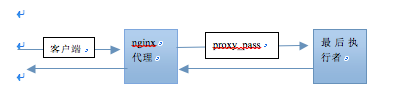三、反向代理的初体验

我们先来实现一个小小的反向代理演示，初步感受一下。小编拿两个域名给大家演示一下，一个域名没有对应到服务，一个域名有对应的服务。

实现效果：当我们访问没有对应服务的域名，然后交给有对应服务的域名进行处理。（如果没有域名的小伙伴，可以通过ip+端口号进行学习）

假设：没有对应服务的域名：http://www.qing48.cn/ ， 有对应服务的域名http://www.battions.com

以上两个域名已过期，抱歉！！！

实现步骤 ：

1、首先将我们http://www.qing48.cn/ 这个域名需要解析到我们对应到Nginx服务上端口是对应到80；

2、结合我们之前学习配置多个虚拟主机的基础之上，配置一个相对应的虚拟主机。

3、在使用Nginx反向代理proxy_pass转发到对应的http://www.battions.com/域名上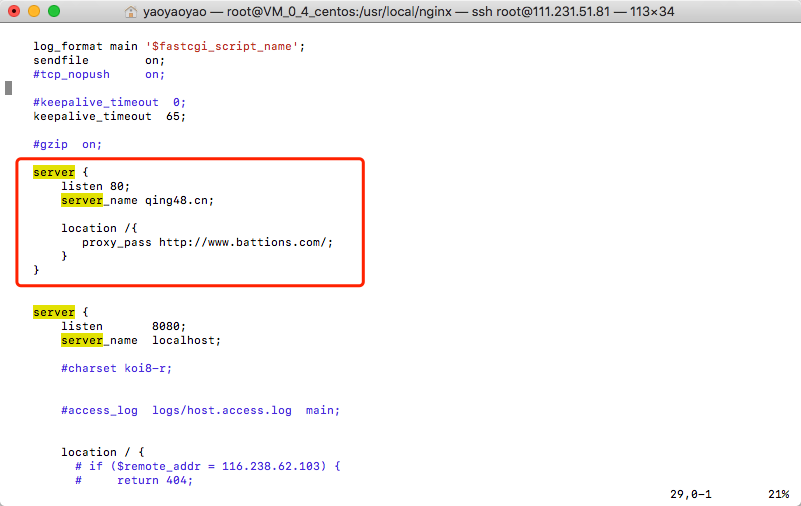四、本章只是带大家初步体验，下个章节会讲如何使用Nginx的反向代理+负载均衡结合的使用！！！

Nginx的反向代理+负载均衡结合教程：点击查看

展开全文负载均衡
• 反向代理：就是从A站配置到B服务器站 反向代理：就是在B站服务器基础上再分到C服务器站 1.设置httpd.conf 打开Apache24/conf文件夹下的httpd.conf设置文件，找到一下几行把前面的注释‘#’删除 ...


一级反向代理：就是从A站配置到B服务器站

二级反向代理：就是在B站服务器基础上再分到C服务器站

1.设置httpd.conf

打开Apache24/conf文件夹下的httpd.conf设置文件，找到一下几行把前面的注释‘#’删除

然后找到
Include conf/extra/httpd-vhosts.conf
这一行前面的注释‘#’也删除，引入这个文件

apache配置一级反向代理，在a站服务器上进行配置

<VirtualHost *:80>

ServerName 您的A站域名

ProxyPassMatch ^/news(.*)$b站ip:b站端口/news$1

ProxyPassMatch ^/news(.*)/$b站ip:b站端口/news$1
ProxyPass /news b站ip:b站端口/news
ProxyPassReverse /news b站ip:b站端口/news

</VirtualHost>

apache在一级反向代理的基础上配置二级反向代理，在b站服务器上进行配置

<VirtualHost *:80>

ServerName 您的b站ip

ProxyPassMatch ^/newsaa(.*)$c站ip:c站端口/newsaa$1

ProxyPassMatch ^/newsaa(.*)/$c站ip:c站端口/news$1
ProxyPass /newsaa c站ip:c站端口/newsaa
ProxyPassReverse /newsaa c站ip:c站端口/newsaa

</VirtualHost>

apache还有更多的功能，比如配置多目录反向代理管理等等

以上就是在目录news下分级newsaa到c服务器的实现配置，未来智库（https://www.7428.cn）提供帮助和二级目录程序服务。  https://www.7428.cn/fml/fml.html


展开全文• ## 反向传播——通俗易懂

万次阅读 多人点赞 2018-10-04 12:05:05
最近在看深度学习的东西，开始看的吴恩达...反向传播法其实是神经网络的基础了，但是很多人在学的时候总是会遇到一些问题，或者看到大篇的公式觉得好像很难就退缩了，其实不难，就是个链式求导法则反复用。如果...
最近在看深度学习的东西，一开始看的吴恩达的UFLDL教程，有中文版就直接看了，后来发现有些地方总是不是很明确，又去看英文版，然后又找了些资料看，才发现，中文版的译者在翻译的时候会对省略的公式推导过程进行补充，但是补充的又是错的，难怪觉得有问题。反向传播法其实是神经网络的基础了，但是很多人在学的时候总是会遇到一些问题，或者看到大篇的公式觉得好像很难就退缩了，其实不难，就是一个链式求导法则反复用。如果不想看公式，可以直接把数值带进去，实际的计算一下，体会一下这个过程之后再来推导公式，这样就会觉得很容易了。

说到神经网络，大家看到这个图应该不陌生：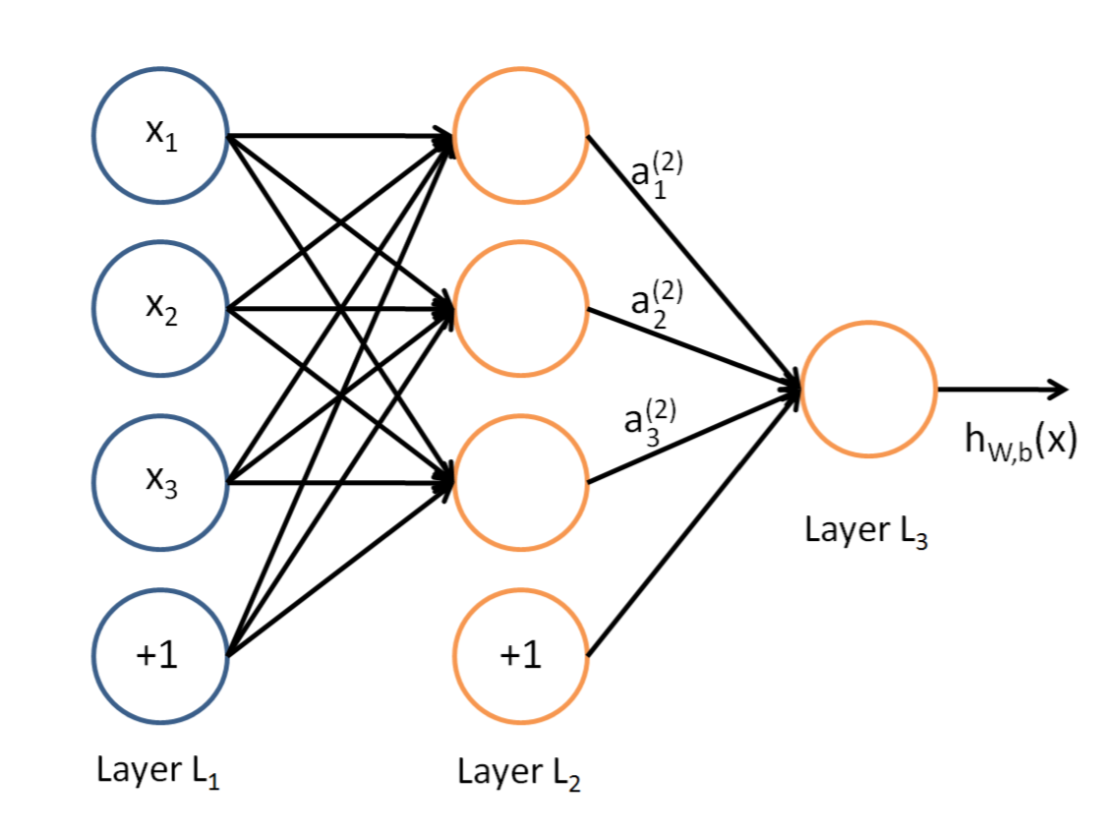这是典型的三层神经网络的基本构成，Layer L1是输入层，Layer L2是隐含层，Layer L3是隐含层，我们现在手里有一堆数据{x1,x2,x3,...,xn},输出也是一堆数据{y1,y2,y3,...,yn},现在要他们在隐含层做某种变换，让你把数据灌进去后得到你期望的输出。如果你希望你的输出和原始输入一样，那么就是最常见的自编码模型（Auto-Encoder）。可能有人会问，为什么要输入输出都一样呢？有什么用啊？其实应用挺广的，在图像识别，文本分类等等都会用到，我会专门再写一篇Auto-Encoder的文章来说明，包括一些变种之类的。如果你的输出和原始输入不一样，那么就是很常见的人工神经网络了，相当于让原始数据通过一个映射来得到我们想要的输出数据，也就是我们今天要讲的话题。

本文直接举一个例子，带入数值演示反向传播法的过程，公式的推导等到下次写Auto-Encoder的时候再写，其实也很简单，感兴趣的同学可以自己推导下试试：）

假设，你有这样一个网络层：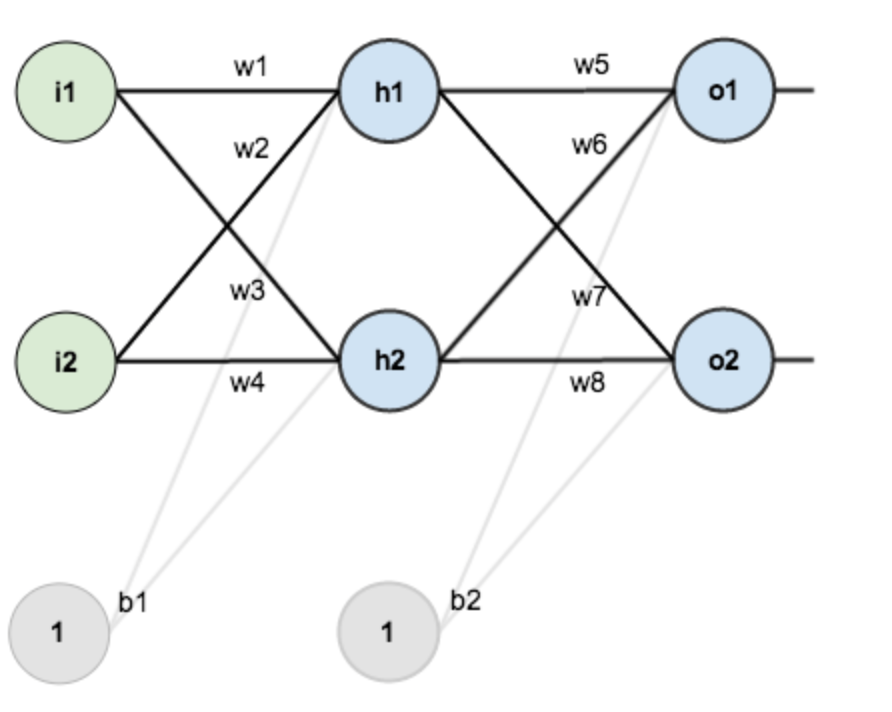第一层是输入层，包含两个神经元i1，i2，和截距项b1；第二层是隐含层，包含两个神经元h1,h2和截距项b2，第三层是输出o1,o2，每条线上标的wi是层与层之间连接的权重，激活函数我们默认为sigmoid函数。

现在对他们赋上初值，如下图：其中，输入数据  i1=0.05，i2=0.10;

输出数据 o1=0.01,o2=0.99;

初始权重  w1=0.15,w2=0.20,w3=0.25,w4=0.30;

w5=0.40,w6=0.45,w7=0.50,w8=0.55

目标：给出输入数据i1,i2(0.05和0.10)，使输出尽可能与原始输出o1,o2(0.01和0.99)接近。

Step 1 前向传播

1.输入层---->隐含层：

计算神经元h1的输入加权和：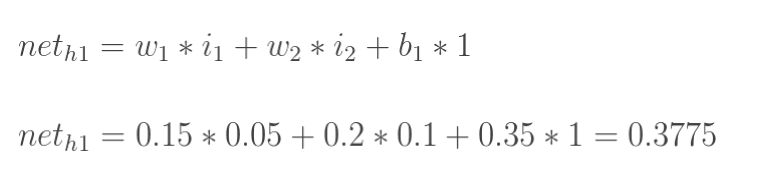神经元h1的输出o1:(此处用到激活函数为sigmoid函数)：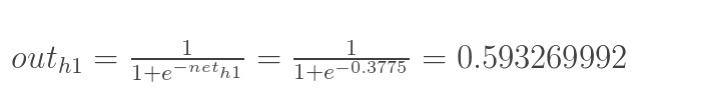同理，可计算出神经元h2的输出o2：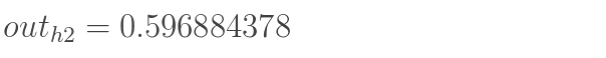2.隐含层---->输出层：

计算输出层神经元o1和o2的值：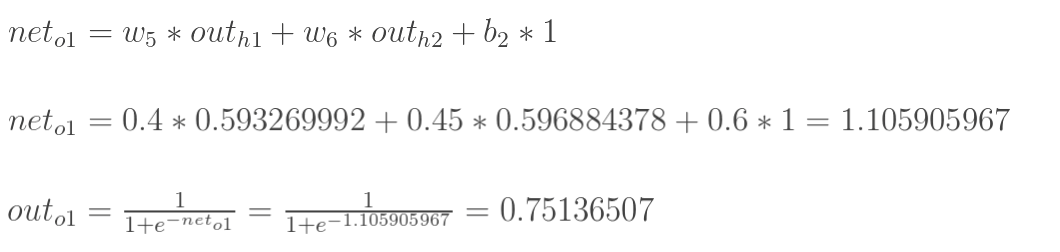这样前向传播的过程就结束了，我们得到输出值为[0.75136079 , 0.772928465]，与实际值[0.01 , 0.99]相差还很远，现在我们对误差进行反向传播，更新权值，重新计算输出。

Step 2 反向传播

1.计算总误差

总误差：(square error)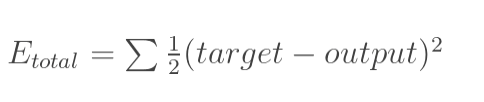但是有两个输出，所以分别计算o1和o2的误差，总误差为两者之和：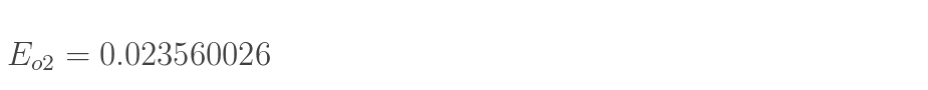2.隐含层---->输出层的权值更新：

以权重参数w5为例，如果我们想知道w5对整体误差产生了多少影响，可以用整体误差对w5求偏导求出：（链式法则）下面的图可以更直观的看清楚误差是怎样反向传播的：现在我们来分别计算每个式子的值：

计算：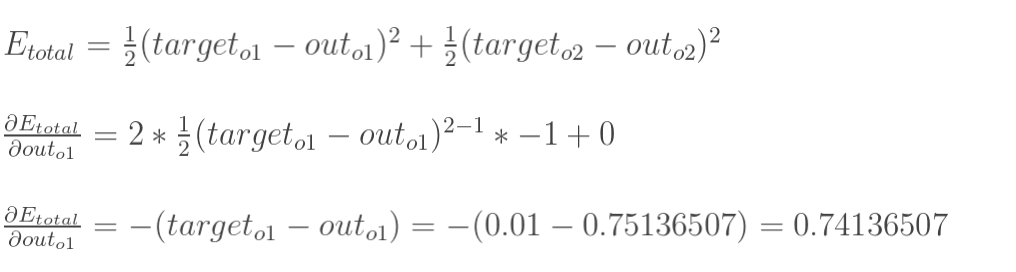计算：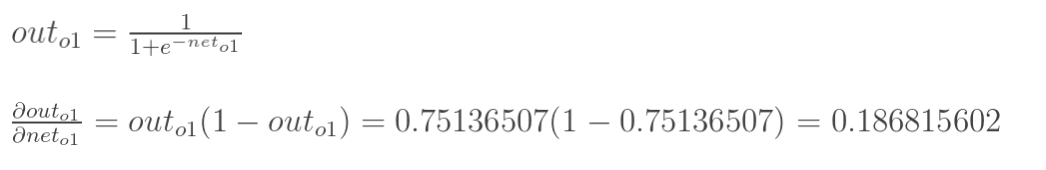（这一步实际上就是对sigmoid函数求导，比较简单，可以自己推导一下）

计算：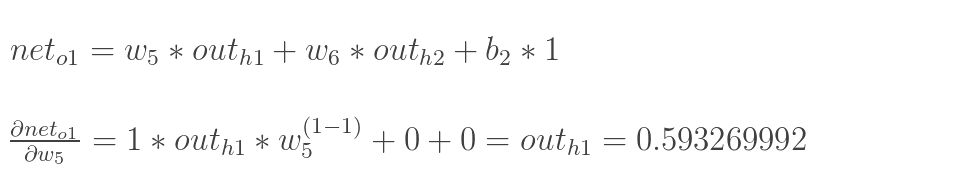最后三者相乘：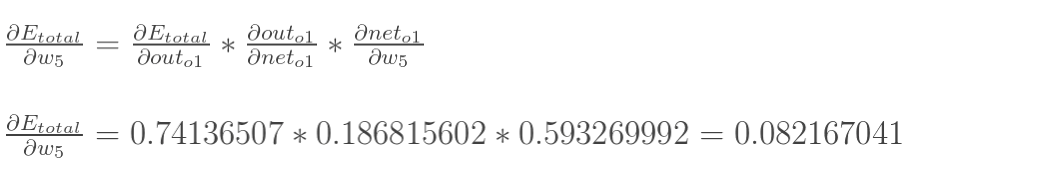这样我们就计算出整体误差E(total)对w5的偏导值。

回过头来再看看上面的公式，我们发现：为了表达方便，用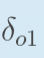来表示输出层的误差：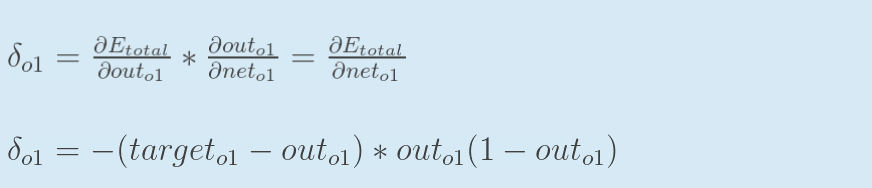因此，整体误差E(total)对w5的偏导公式可以写成：如果输出层误差计为负的话，也可以写成：最后我们来更新w5的值：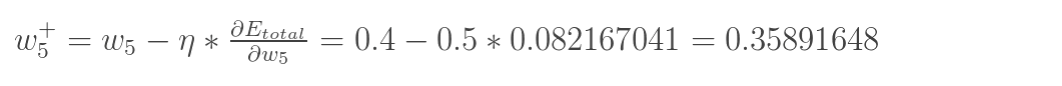（其中，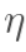是学习速率，这里我们取0.5）

同理，可更新w6,w7,w8: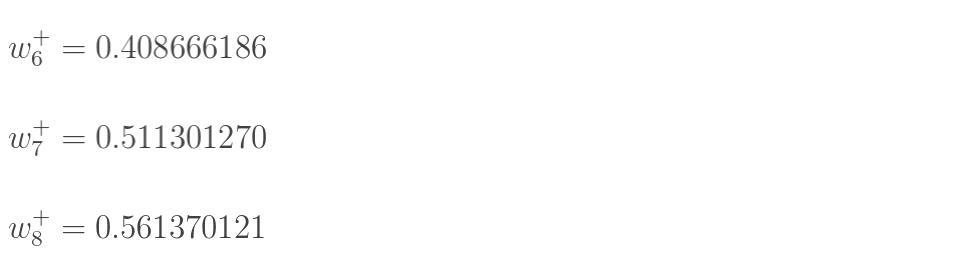3.隐含层---->隐含层的权值更新：

方法其实与上面说的差不多，但是有个地方需要变一下，在上文计算总误差对w5的偏导时，是从out(o1)---->net(o1)---->w5,但是在隐含层之间的权值更新时，是out(h1)---->net(h1)---->w1,而out(h1)会接受E(o1)和E(o2)两个地方传来的误差，所以这个地方两个都要计算。计算：先计算：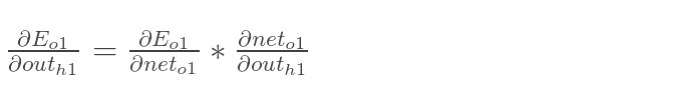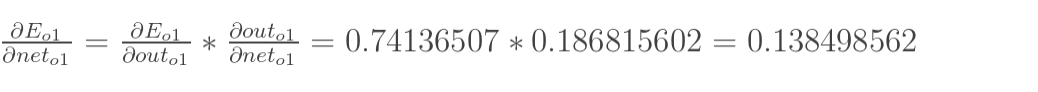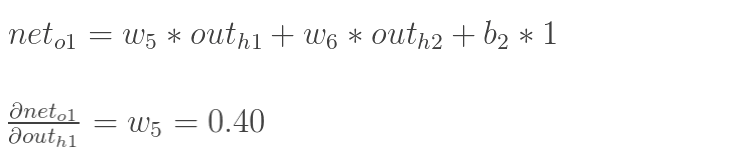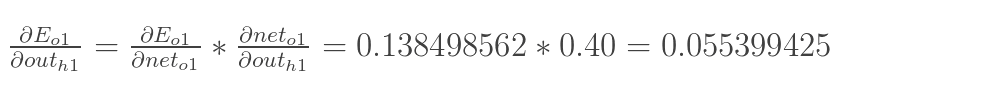同理，计算出：两者相加得到总值：再计算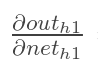：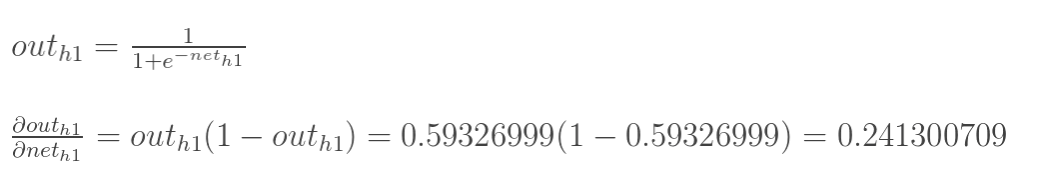再计算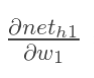：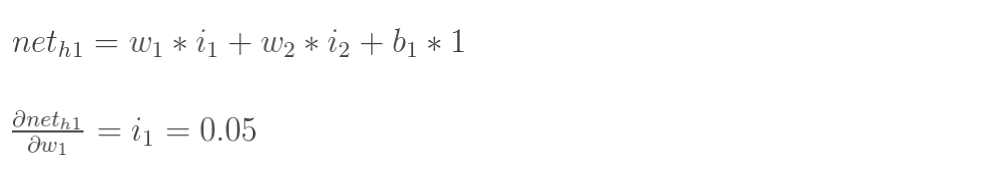最后，三者相乘：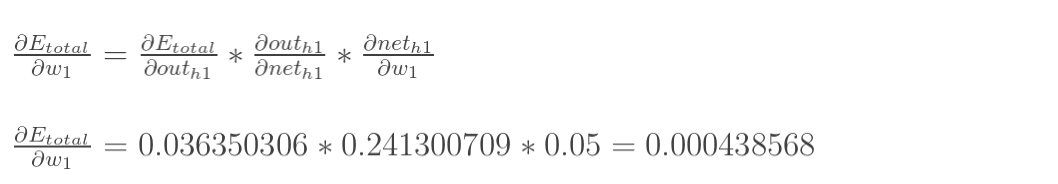为了简化公式，用sigma(h1)表示隐含层单元h1的误差：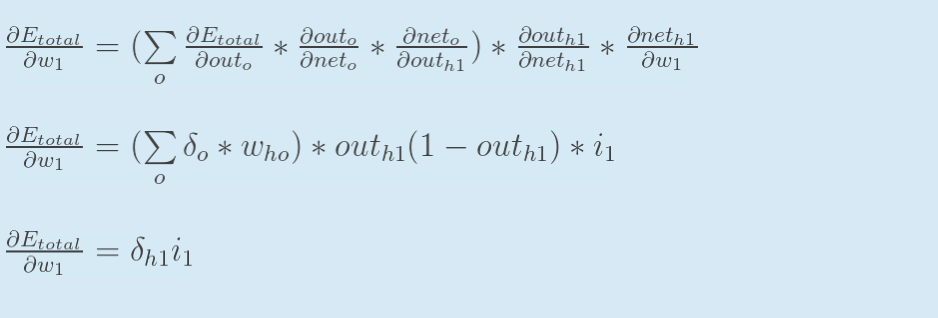最后，更新w1的权值：同理，额可更新w2,w3,w4的权值：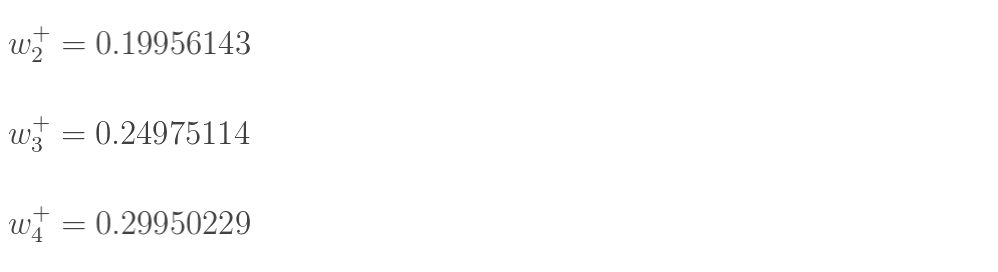这样误差反向传播法就完成了，最后我们再把更新的权值重新计算，不停地迭代，在这个例子中第一次迭代之后，总误差E(total)由0.298371109下降至0.291027924。迭代10000次后，总误差为0.000035085，输出为[0.015912196,0.984065734](原输入为[0.01,0.99]),证明效果还是不错的。

展开全文• ## nginx反向代理配置去除前缀

万次阅读 多人点赞 2019-01-07 00:13:28
使用nginx做反向代理的时候，可以简单的直接把请求原封不动的转发给下个服务。设置proxy_pass请求只会替换域名，如果要根据不同的url后缀来访问不同的服务，则需要通过如下方法： 方法：加"/" server ...nginx
• ## Nginx反向代理

千次阅读 2020-12-24 19:02:22
文章目录Nginx系列文章目录nginx介绍nginx正向代理介绍反向代理简介反向代理原理三、反向代理作用总结 nginx介绍 nginx介绍：https://blog.csdn.net/qq_45937199/article/details/111637912 nginx正向代理...nginx
• #反向代理# ##百科 反向代理服务器决定哪台服务器提供服务。 ...##Nginx实现反向代理 ...两个域名指向同一台nginx服务器，...第步：安装两个tomcat，分别运行在8080和8081端口。 第步：启动两个tomcat。 ...
• 文章目录、为什么引入反向传播反向传播的重要性三、反向传播的推导过程四、反向传播与梯度下降的关系 、为什么引入反向传播 神经网络的过程就是正向传播得到Loss值，再把Loss值反向传播，并对神经网络的参数...
• ## nginx反向代理--负载均衡

万次阅读 多人点赞 2018-10-19 17:39:37
nginx是款自由的、开源的、高性能的HTTP服务器和反向代理服务器；同时也是个IMAP、POP3、SMTP代理服务器；nginx可以作为个HTTP服务器进行网站的发布处理，另外nginx可以作为反向代理进行负载均衡的实现。 ...
• ## Nginx实现反向代理

千次阅读 2018-11-06 17:12:04
、什么是反向代理？ 反向代理（Reverse Proxy）方式是指以代理服务器来接受internet上的连接请求，然后将请求转发给内部网络上的服务器，并将从服务器上得到的结果返回给internet上请求连接的客户端，此时代理...
• ## 反向telnet

千次阅读 2013-07-10 09:45:10
反向telnet 路由器异步线路与Modem连接后，就可以建立与Modem的直接Telnet对话通信。这个过程也称为反向远程登录Telnet( reverse Telnet)。 反向远程登录的意思是经由异步线路启动Telnet对话,而不是接收传至线路...
• 最近在做个RPF 反向供电的项目。 反向供电的FTTDp,主要市场还是欧美，澳洲等传统的DSL铜线国家。现在在铜线上已经有比较成熟的GFast和正在实验室开发的xGFast。 G.Fast的总带宽可以到 1Gbps,其频谱宽度为106MHz...
• J2EE进阶(十五)MyEclipse反向工程实现从数据库反向生成实体类之Hibernate方式  反向工程又称逆向工程。   开发项目涉及到的表太多，个的写JAVA实体类很是费事。MyEclipse提供简便的方法：反向数据库。 ...hibernate myeclipse java 数据库
• ## NGINX配置反向代理

千次阅读 2020-10-09 15:51:11
章 2.1-2.2 利用NGINX配置反向代理,认识正向代理与反向代理,以及Nginx反向代理location的匹配规则nginx linux 正向代理 服务器
• 本文以MySQL为例，简述一下PD反向工程的步骤： 、配置 JDK 环境变量 首先，我是用的 PowerDesigner 版本是 16.5.0.3982 截止目前，PD反向工程仅支持32位的jdk（重点！重点！...、配置 PowerDes...
• 反向代理服务器位于用户与目标服务器之间，但是对于用户而言，反向代理服务器就相当于目标服务器，即用户直接访问反向代理服务器就可以获得目标服务器的资源。同时，用户不需要知道目标服务器的地址，也无须在用户端...
• ## 反向解析RDNS

千次阅读 2018-11-13 09:22:24
• 、为啥要叫反向代理 正向代理隐藏真实客户端，反向代理隐藏真实服务端。 反向代理解决的问题 反向代理解决的就是跨域，在前后端联调的情况下，前端有服务器，后端也有服务器，那么联调时，就存在跨域问题...
• ## squid反向代理

千次阅读 2020-02-27 15:18:14
当用户浏览器发出个HTTP请求时，通过域名解析将请求定向到反向代理服务器（如果要实现多个WEB服务器的反向代理，需要将多个WEB服务器的域名都指向反向代理服务器）。由反向代理服务器处理器请求。反向代理一般只...
• 、准备工作 Linux系统安装Tomcat，使用默认端口8080，启动...反向代理配置 在Windows系统的host文件进行域名和IP地址映射关系的配置 可以通过域名8080端口访问到Tomcat服务器 然后在Nginx进行请求转发的配Nginx
• 我原来用的是sql2000，用powerdesigner12反向生成pdm物理数据模型，一切正常，驱动调到sql2000. 最近升级数据库到sql2005，再反向时，驱动调到sql2005，但是生成时总报错： "Unable to list the tables. ...sql sql server microsoft 数据库 list
• 反向原理 基于交易市场的“二八定律”，即“盈八亏”或“一盈平七亏”，依据市场的交易行为，进行反方向操作，锁定盈利。 ．反跟途径 运用计算机技术，搭建一套虚拟交易端软件，连接时时行情数据，再搭建...搜索引擎
• ## 反向DNS查询

千次阅读 2018-04-01 16:56:24
在计算机网络中，反向DNS查询或反向DNS解析（rDNS）是查询域名系统（DNS）以...建议“每个可访问Internet的主机都应该有个名称”，并且“对于每个IP地址，应该有个匹配的PTR记录”，但这不是Internet标准的要求......